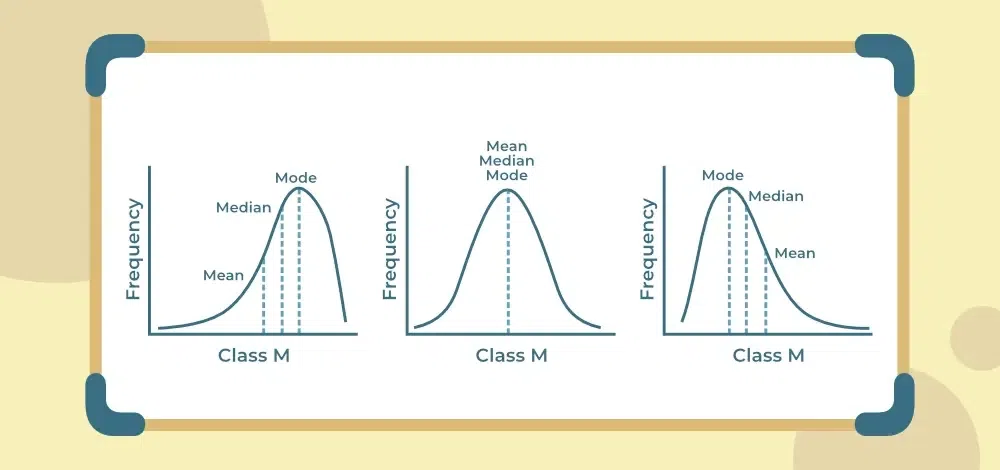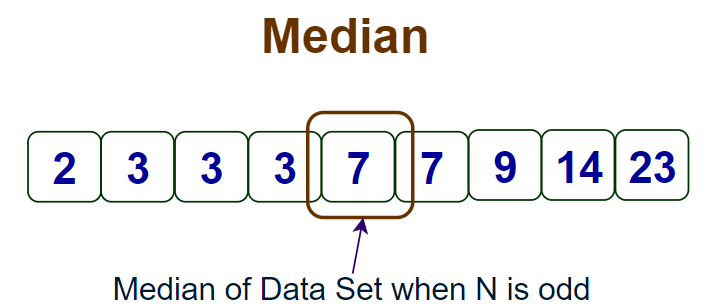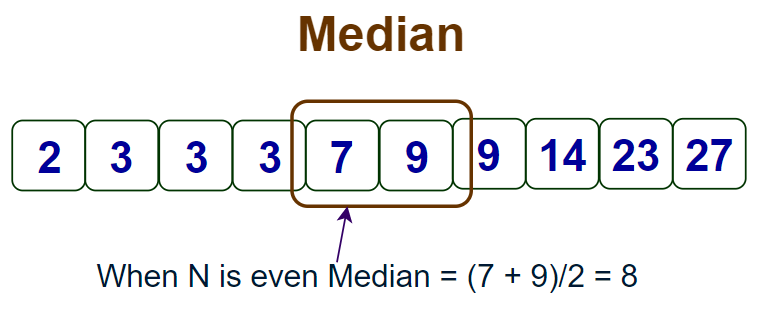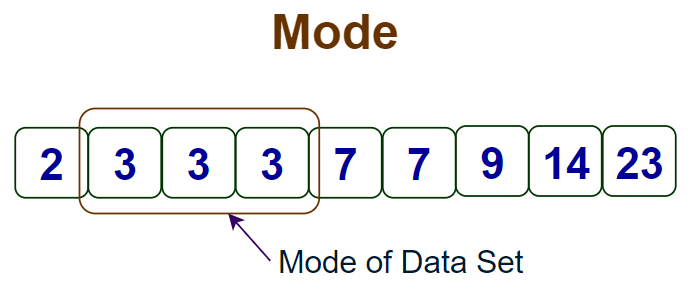Open In App

# Measures of Central Tendency in Statistics

Central Tendencies in Statistics are the numerical values that are used to represent mid-value or central value a large collection of numerical data. These obtained numerical values are called central or average values in Statistics. A central or average value of any statistical data or series is the value of that variable that is representative of the entire data or its associated frequency distribution. Such a value is of great significance because it depicts the nature or characteristics of the entire data, which is otherwise very difficult to observe.

## Measures of Central Tendency Meaning

The representative value of a data set, generally the central value or the most occurring value that gives a general idea of the whole data set is called the Measure of Central Tendency.

## Measures of Central Tendency

Some of the most commonly used measures of central tendency are:

• Mean
• Median
• Mode## Mean

Mean in general terms is used for the arithmetic mean of the data, but other than the arithmetic mean there are geometric mean and harmonic mean as well that are calculated using different formulas. Here in this article, we will discuss the arithmetic mean.

### Mean for Ungrouped Data

Arithmetic mean () is defined as the sum of the individual observations (xi) divided by the total number of observations N. In other words, the mean is given by the sum of all observations divided by the total number of observations.OR

Mean = Sum of all Observations ÷ Total number of Observations

Example: If there are 5 observations, which are 27, 11, 17, 19, and 21 then the mean () is given by= (27 + 11 + 17 + 19 + 21) ÷ 5

⇒= 95 ÷ 5

⇒= 19

### Mean for Grouped Data

Mean () is defined for the grouped data as the sum of the product of observations (xi) and their corresponding frequencies (fi) divided by the sum of all the frequencies (fi).Example: If the values (xi) of the observations and their frequencies (fi) are given as follows:

 xi fi 4 6 15 10 9 5 10 8 7 10

then Arithmetic mean () of the above distribution is given by= (4×5 + 6×10 + 15×8 + 10×7 + 9×10) ÷ (5 + 10 + 8 + 7 + 10)

⇒= (20 + 60 + 120 + 70 + 90) ÷ 40

⇒= 360 ÷ 40

⇒= 9

Related Resources,

### Types of Mean

Mean can be classified into three different class groups which are

• Arithmetic Mean
• Geometric Mean
• Harmonic Mean

Arithmetic Mean: The formula for Arithmetic Mean is given byWhere,

• x1, x2, x3, . . ., xn are the observations, and
• N is the number of observations.

Geometric Mean: The formula for Geometric Mean is given byWhere,

• x1, x2, x3, . . ., xn are the observations, and
• n is the number of observations.

Harmonic Mean: The formula for Harmonic Mean is given byORWhere,

• x1, x2, . . ., xn are the observations, and
• n is the number of observations.

### Properties of Mean (Arithmetic)

There are various properties of Arithmetic Mean, some of which are as follows:

• The algebraic sum of deviations from the arithmetic mean is zero i.e.,.
• Ifis the arithmetic mean of observations and a is added to each of the observations, then the new arithmetic mean is given by• Ifis the arithmetic mean of observations and a is subtracted from each of the observations, then the new arithmetic mean is given by• Ifis the arithmetic mean of observations and a is multiplied by each of the observations, then the new arithmetic mean is given by• Ifis the arithmetic mean of observations and each of the observations is divided by a, then the new arithmetic mean is given by### Disadvantage of Mean as Measure of Central Tendency

Although Mean is the most general way to calculate the central tendency of a dataset however it can not give the correct idea always, especially when there is a large gap between the datasets.

## Median

The Median of any distribution is that value that divides the distribution into two equal parts such that the number of observations above it is equal to the number of observations below it. Thus, the median is called the central value of any given data either grouped or ungrouped.

### Median of Ungrouped Data

To calculate the Median, the observations must be arranged in ascending or descending order. If the total number of observations is N then there are two cases

Case 1: N is Odd

Median = Value of observation at [(n + 1) ÷ 2]th Position

When N is odd the median is calculated as shown in the image below.Case 2: N is Even

Median = Arithmetic mean of Values of observations at (n ÷ 2)th and [(n ÷ 2) + 1]th Position

When N is even the median is calculated as shown in the image below.Example 1: If the observations are 25, 36, 31, 23, 22, 26, 38, 28, 20, 32 then the Median is given by

Arranging the data in ascending order: 20, 22, 23, 25, 26, 28, 31, 32, 36, 38

N = 10 which is even then

Median = Arithmetic mean of values at (10 ÷ 2)th and [(10 ÷ 2) + 1]th position

⇒ Median = (Value at 5th position + Value at 6th position) ÷ 2

⇒ Median = (26 + 28) ÷ 2

⇒ Median = 27

Example 2: If the observations are 25, 36, 31, 23, 22, 26, 38, 28, 20 then the Median is given by

Arranging the data in ascending order: 20, 22, 23, 25, 26, 28, 31, 36, 38

N = 9 which is odd then

Median = Value at [(9 + 1) ÷ 2]th position

⇒ Median = Value at 5th position

⇒ Median = 26

### Median of Grouped Data

The Median of Grouped Data is given as follows:Where,

• l is the lower limit of median class,
• n is the total number of observations,
• cf is the cumulative frequency of the preceding class,
• f is the frequency of each class, and
• h is the class size.

Example: Calculate the median for the following data.

 Class Frequency 10 – 20 20 – 30 30 – 40 40 – 50 50 – 60 5 10 12 8 5

Solution:

Create the following table for the given data.

ClassFrequencyCumulative Frequency

10 – 20

5

5

20 – 30

10

15

30 – 40

12

27

40 – 50

8

35

50 – 60

5

40

As n = 40 and n/2 = 20,

Thus, 30 – 40 is the median class.

l = 30, cf = 15, f = 12, and h = 10

Putting the values in the formulaMedian = 30 + (20 – 15)/12) × 10

⇒ Median = 30 + (5/12) × 10

⇒ Median = 30 + 4.17

⇒ Median = 34.17

So, the median value for this data set is 34.17

## Mode

The Mode is the value of that observation which has a maximum frequency corresponding to it. In other, that observation of the data occurs the maximum number of times in a dataset.

### Mode of Ungrouped Data

Mode of Ungrouped Data can be simply calculated by observing the observation with the highest frequency. Let’s see an example of the calculation of the mode of ungrouped data.

The mode of the data set is the highest frequency term in the data set as shown in the image added below.Example: Find the mode of observations 5, 3, 4, 3, 7, 3, 5, 4, 3.

Solution:

Create a table with each observation with its frequency as follows:

 xi fi 5 3 4 7 2 4 2 1

Since 3 has occurred a maximum number of times i.e. 4 times in the given data;

Hence, Mode of the given ungrouped data is 3.

### Mode of Grouped Data

The formula to find the mode of the grouped data is:Where,

• l is the lower class limit of modal class,
• h is the class size,
• f1 is the frequency of modal class,
• f0 is the frequency of class which proceeds the modal class, and
• f2 is the frequency of class which succeeds the modal class.

Example: Find the mode of the dataset which is given as follows.

 Class Interval Frequency 10-20 20-30 30-40 40-50 50-60 5 8 12 16 10

Solution:

As the class interval with the highest frequency is 40-50, which has a frequency of 16. Thus, 40-50 is the modal class.

Thus, l  = 40 , h = 10 , f1 = 16 , f0 = 12 , f2 = 10

Plugging in the values in formula, we get

Mode = 40 + (16 – 12)/(2 × 16 – 12 – 10) × 10

⇒ Mode = 40 + (4/10)×10

⇒ Mode = 40 + 4

⇒ Mode = 44

Therefore, the mode for this set of data is 44.

### Empirical Relation Between Measures of Central Tendency

The three central tendencies are related to each other by the empirical formula which is given as follows:

2 × Mean + Mode = 3 × Median

This formula is used to calculate one of the central tendencies when two other central tendencies are given.

## FAQs on Measures of Central Tendency

### 1. What is a Measure of Central Tendency in Statistics?

Measure of Central Tendency of a dataset represent a central value or a typical value for a dataset which can be used to do further analysis on the data.

### 2. What is the Mean?

Mean is the Average value of the dataset and can be calculated Arithmetically, Geometrically, and Harmonically as well. Generally by term “mean” means the arithmetic mean of the data.

### 3. When is the Mean a good measure of Central Tendency?

Mean is a good measure of central tendency when data is normally distributed and there is no extreme values or outliers in the dataset.

### 4. What is the Median?

Median is the middle value of the data set when arranged in increasing or decreasing order i.e., in either ascending or desending order there are equal number of observations on both sides of median.

### 5. When is the Median a good measure of Central Tendency?

The median is a good measure of central tendency when dataset is skewed or there are extreme values or outliers in the dataset.

### 6. What is the Mode?

Mode is highest frequency observation of the given dataset.

### 7. When is the Mode a good measure of Central Tendency?

The mode is a good measure of central tendency when there are clear peaks in the dataset of frequencies of observations.

### 8. Can a dataset have more than one mode?

Yes, a dataset can have more than one mode as there can be two observations with same number of highest frequencies.

### 9. What is the purpose of central tendency?

The primary goal of central tendency is to offer a single value that effectively represents a set of collected data. This value aims to capture the core or typical aspect of the data, providing a concise summary of the overall information.